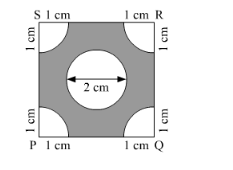# In the following figure,

Question:

In the following figure, PQRS is a square of side 4 cm. Find the area of the shaded square.Solution:

Area of the shaded region is equal to the area of the square minus area of four sectors with the same radius minus area of the circle.

We know that area of the four sectors with radius is equal to area of one circle.

$\therefore$ Area of the shaded region $=4^{2}-\pi \times 1^{2}-\pi \times 1^{2}$

$\therefore$ Area of the shaded region $=4^{2}-2 \times \pi \times 1$

$\therefore$ Area of the shaded region $=16-2 \pi$

Therefore, area of the shaded region is $(16-2 \pi) \mathrm{cm}^{2}$